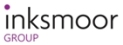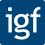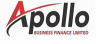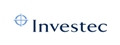• # How To Calculate Discount Charge FeesHow to calculate discount charge fees can be one of the most complicated parts of using a factoring, invoice finance or invoice discounting facility. Below we explain exactly how these charges work.

#### Invoice Finance Charges

The discount fee may be just one part of pricing structure of your facility. Typically, there will be a service charge, discount fee and other charges. These are explained in our article: How Pricing Works.

With some types of pricing there may not be a separate discount element, this is called "single fee" pricing.

This article just explains how the discount element works, if it is present.

You can see which charges are applicable, and what they are, on your offer letter or facility agreement.

#### How To Calculate Discount Charge

Discount charge (DC) is technically not interest, although the calculations are very similar. The discount is charged in respect of the amount of funds that are outstanding at any given time.

Most funders work by charging for the number of days that funds are in use. So every day DC will accrue in respect of the balance outstanding - this is often called the "current account", or "funds in use" (FIU).

The DC is made up of two elements, the base and the margin. The base is normally base rate (BR - from a specified bank - typically the one used by the funder) and the discount margin (DM). In some cases LIBOR is used instead of BR, but the numbers work in exactly the same way - LIBOR can just be a different value to base rate. So the DC will often be quoted, for example as 2% over base. That means the DM is 2% and base will be whatever value it is at the time that they charge is calculated.

BEWARE: some funders use a minimum base rate. If that minimum was set at say 2.5%, even if base rate drops to 0.1%, you still pay 2.5% (plus the DM)! Most funders do not do this.

DC works the same for all types of invoice finance facility.

#### Discount Fee Calculation

To calculate the discount charge use the following formula (remember to adjust for any minimum base rate):

• Discount charge = ((FIU x (DM + BR)) / 365) x number of days.

The division by 365 days will be adjusted for leap years but please note that some funders use different divisors e.g. 360 days. This will marginally increase the discount charged. The "number of days" is the number of days those funds were outstanding. Funder's systems will tend to calculate discount on a daily basis.

To describe that calculation, you are adding together the discount margin plus base rate percentages, and multiplying the funds in use by that percentage. This gives you the amount of discount accrued each year. You then divide by the number of days in a year, to get the daily discount. You then multiply by the number of days the funds are outstanding in order to calculate the discount fee.

This is a worked example using FIU of £100,000, DM of 2.5%, BR of 0.1%, outstanding for 30 days:

• Discount charge = ((100,000 x (2.5% + 0.1%)) / 365) x 30
• Discount fee = £213.70

#### Variations Between Invoice Finance Companies

The calculation is standard across funders, although different values will be used:

• Different base rate values by employing minimums
• Different discount margins may be quoted
• Different numbers of days per annum may be used

We are always happy to help you check and calculate the discount charge from your statements of account.

I have read and accept your:
Customer Terms and Conditions.

Examples of just a few of our finance partners: